telescopeѲptics.net          ▪▪▪▪                                             CONTENTS

# 8.4.2. Two-mirror tilted component telescopes

By replacing the flat in a Herschelian with a toroid, in the same configuration, or with a second curved mirror directing light back toward primary, needed mirror separation can be reduced roughly two two three times, for a similar level of correction. Field asymmetry is also reduced. Best known systems of this type of tilted component telescopes (TCT) are the variants Schiefspiegler and Yolo.

The former is introduced by S.A. Leonard in the early 1960s. It uses two concave mirrors, one of them toroidal (a sphere deformed into a toroid by a specially designed cell). In general, it achieves better - and exceptionally good - performance than the Schiefspiegler (Schief for short), and the systems can be considerably faster. On the other hand, it is also more complex. The Schief is created by Anton Cutter, and originally uses a pair of spherical mirrors, the secondary being convex. The aberration here is present in the field center, and has to be kept acceptably low by limiting a system to quite small relative apertures, typically ~f/20 and smaller.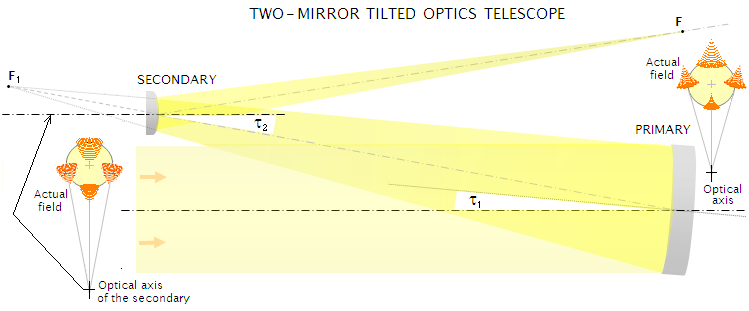FIGURE 134: The two-mirror TCT final field forms as a combination of the effective field at each mirror. Since the combining fields are each a circular off axis area of mirror's field, they are not symmetric about their respective center points. Since they are likely to have not only different magnitude of astigmatism, but also different ratio of coma vs. astigmatism - each changing differently with the field angle - once the aberration of their combined centerfield point is minimized with a given combination of tilt angles, aberration distribution over the field is more or less asymmetrical.

Aberrations of a tilted-mirror system are a sum of the aberrations at each mirror. The principles of aberration compensation can be outlined using the basic two-mirror arrangement. Here, aberrations at the primary are simply off-axis aberrations for the field angle equal to a mirror tilt angle τ1, typically ~3°, and possibly residual spherical aberration to compensate for that induced by the secondary (not needed in traditional TCT arrangements, due to mild surface curvatures).

For the secondary, the object is the image projected by the primary. Since the secondary is centered around the reflected axis of the primary (i.e. around the chief ray of the axial cone), its aperture stop coincides with its surface for the center-field aberrations, not only for spherical aberration, but also for center-field astigmatism and coma. For other field points, the chief ray height at the secondary differs from zero, and the aperture stop is shifted to the primary.

These features make two-mirror TCT alike axial two-mirror systems in one, and different in another respect. The similarity extends to secondary magnification and spherical aberration, for which the same general two-mirror system relations (Eq. 78 and Eq. 80, respectively) apply to TCT's as well, including center-field astigmatism and coma, formally abaxial aberrations. For TCT's abaxial aberrations outside the field center, regular stop-shift relations for abaxial aberrations of the two-mirror system secondary apply.

This means that for astigmatism wavefront error, which doesn't change with object distance, and for coma, which changes with object distance, the aberration relations that apply for the secondary mirror contribution in the field center are Eq. 19, and Eq. 15, respectively. For all other field points, astigmatism and coma at the secondary are calculated either from general two-mirror system relations (Eq. 82-82.1), or from Eq. 15.2-15.3 and Eq. 19.1 and Eq. 22 for coma and astigmatism, respectively.

The consequence of TCT mirror tilt is that it places off-axis aberrations in the field center by effectively moving the field far off-axis - with individual aberration contributions of the primary and secondary being determined by their tilt angles. The primary mirror field is a circle far off from the optical axis (left on the above illustration), with strong astigmatism and coma at the same level along the field cross-radius centered at the optical axis (the field center for axially oriented mirror). Along the central field meridian orthogonal to this radius, coma and astigmatism increase according to the angle measured from the optical axis. In between, there is a gradual transition from one form of aberrated field to another. Actual TCT field radius is typically only a fraction of the tilt angle at the primary.

TCT's primary mirror center field astigmatism and coma, commonly at the level of 10 and 2-3 waves P-V, or higher (respectively) are, for best focus location, given by:

Wa1=-D1(τ1+α)2/8F      and      Wc1=(τ1+α)D1/48F2

respectively, with D1 being the aperture diameter, α the angular differential, in radians, between the tilt angle τ1 and the angle determined by the point distance from the optical axis (for the tangential - vertical - field meridian, it corresponds to field angle relative to the field center), and F the focal ratio of the primary.

The main goal of the two-mirror TCT's secondary is to minimize this center-field aberration induced by tilted primary. This is accomplished by tilting it to induce offsetting aberrations, with the secondary mirror tilt angle τ2 measured with respect to the axial ray reflected from the primary (i.e. the chief ray for axial cone). Center-field astigmatism and coma at the secondary, also as the P-V wavefront error at the best focus location, are given by:

Wa2 = -τ22D22/4R2
and
Wc2 = τ2(1-Ω)D23/12R22

respectively, with R2, D2, τ2 being the secondary radius of curvature, effective aperture diameter (i.e. the minimum secondary size) and tilt angle, respectively, α being the field angle as defined earlier, and Ω=R2/l the inverse of relative object distance for the secondary l (secondary to projected focus of the primary separation) in units of the secondary radius of curvature. Since both, R2 and l are, according to the sign convention, numerically negative, the sign of Ω is positive.

Note that only a convex secondary, with numerically negative radius of curvature, offsets astigmatism and coma generated by tilted primary.

For the field points outside the center, astigmatism and coma at the secondary, as the P-V error at the best focus location, are given by: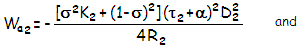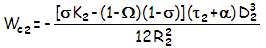with σ being the secondary to the aperture stop (i.e. primary) separation in units of secondary's radius of curvature, numerically negative for convex secondary (note that these relations are valid for two-mirror system secondary in general, with the tilt angle τ=0 for axial systems). The field angle α, as mentioned before, is the angular differential between the tilt angle of the secondary to the reflected optical axis of the primary and field point angle measured from the point to the reflected primary's axis. Numerically, it can be either positive or negative (zero for the field center).

With coma and astigmatism being in different proportions throughout the field of the two mirrors, due to their different tilt angles (that of the secondary is commonly in excess of double the primary's tilt angle), the final image in a simple TCT, after the center-field aberration is minimized, has only partially corrected field - unless of very small relative aperture - with uneven distribution of off-axis aberrations. Uneven compensation of astigmatism can, and most often does induce image tilt. Even the very field center is a compromise: since it is impossible to generate with the secondary's tilt the exact proportion of astigmatism and coma that are induced by primary's tilt, some level of residual aberration remains present.

These consequences of the uneven aberration match of two different far off-axis field segments limit simple two-mirror TCTs - and most more complex tilted systems as well - to small relative apertures and smallish aperture diameters.

EXAMPLE: Anton Kutter's 110mm f/24.7 schiefspiegler, with concave f/14.7 spherical primary and spherical convex secondary, with the primary-to-secondary separation of 965mm. Radii of curvature R1=R2=-3240mm, secondary to primary's projected focus distance l=-655mm, the inverse of relative object distance for the secondary Ω=R2/l=4.95, secondary mirror effective diameter D2=44.5mm, and the tilt angles τ1=2.65° and τ2=6.65° for the primary and secondary mirror, respectively. Best image field is astigmatic but flat.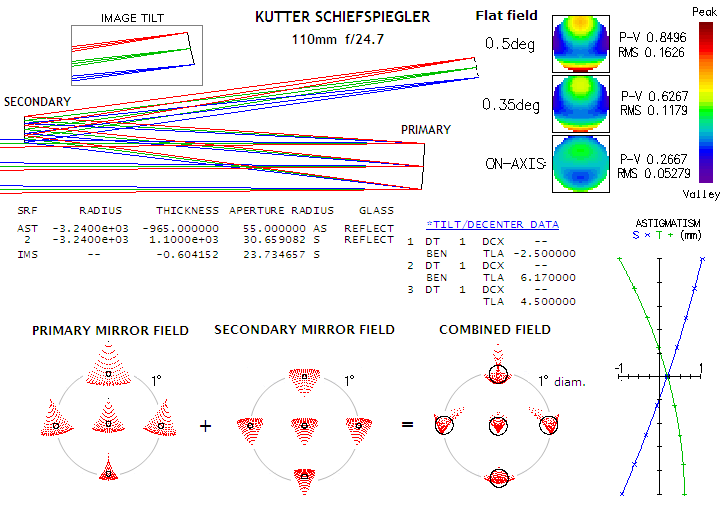In the field center (α=0), primary mirror astigmatism and coma contribution is Wa1=-0.00193mm (3.5 waves P-V, in 550nm wavelength units), and Wc1=0.00048mm (0.9 waves P-V), respectively. With secondary mirror contributions of Wa2=0.00198mm and Wc2=-0.00032mm, the system aberrations are WaS=Wa1+Wc1=0.00005mm, or 0.09 wave P-V of Seidel astigmatism (higher-order is entirely insignificant), and WcS=Wc1+Wc2=0.00016mm, or 0.29 wave P-V of coma. This is close to 0.27 wave P-V output by the exact ray trace (OSLO) for the final center field aberration (relatively low astigmatic deformation has little effect on the P-V error of coma).
This system is titled "anastigmatic" due to the astigmatism being nearly cancelled in the field center; however, there is still strong astigmatism present in the outer field. Center-field coma could be cancelled by tilting the secondary more, to 9.7°, but astigmatism would become unacceptably large. The ray spot plots illustrate the field match in this two-mirror TCT system. Secondary's field is farther away from its axis, thus its ray spots  have larger proportion of astigmatism, lower degree of variation in the size of aberrations across the field's vertical diameter, and less of the angular spot  inclination toward the ends of field along the diameter orthogonal to it. These differences between the two combining field result in the final seemingly disarrayed, asymmetrical field (the plot is for the best image surface, tilted at ~4.5° counterclockwise to the axial ray, as shown in inset top left).
Since the aberrations of two tilted mirrors are a sum of their respective partly offsetting aberrations, their center-field residue will increase in proportion with aperture, but exponentially in inverse proportion to the system focal ratio. For instance, at a twice as fast focal ratio, coma contribution will be four times larger by each mirror due to the radius cut in half, and nearly doubled due to both tilt angles doubled (nearly, because it is in proportion to the sum of tilt angle, and comparably small field angle). As a result, center field coma in f/12.3  system of this aperture would have been nearly eight times larger.
Also, since the field astigmatism here is a residual to the opposite in sign field astigmatism of each mirror, roughly comparable in magnitude, it is not increasing with the square of field angle, as in centered systems, but approximately in proportion to it (the rate varies somewhat with the field radius).
Note that the raytrace indicates that the primary tilt is not quite sufficient to place the secondary out of incoming rays for 0.5
° field angle. Required tilt for that is 2.9°, which would increase the center-field aberration by about 10%, and also slightly
(about 5%) the off axis astigmatism.
Center-field performance in this configuration can be improved making a system catadioptric, by placing a strongly tilted plano-convex lens in the path of light converging from the secondary. The gain is in the center field correction, with the outer field remaining of similar quality for given focal ratio. Longitudinal chromatism is practically non-existent, but lateral color error may not be in larger apertures (~150mm and larger at ~
f/20). As shown below, lateral color is asymmetric, diminishing toward one, while increasing toward the opposite direction. It can be corrected by making the lens slightly wedged. In this case, the wedge angle is 0.049° (2.94 arc minutes); in order to compensate for the change in astigmatism, lens' tilt is slightly reduced.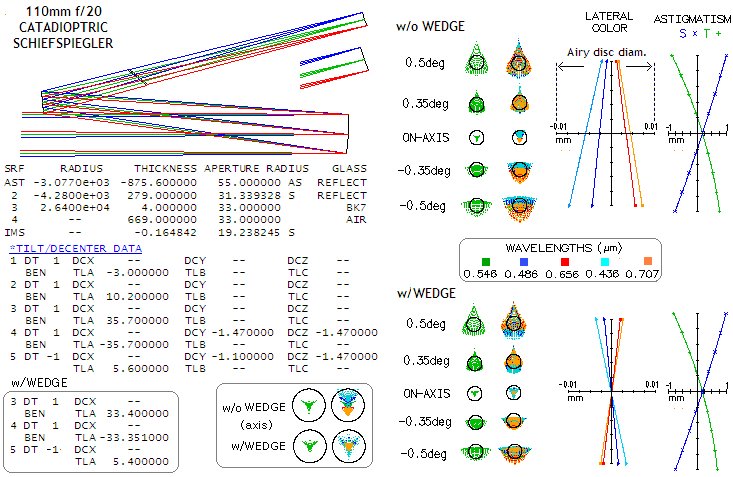Another option is to make the secondary toroid. It allows for better overall correction of aberrations. The secondary can be either convex, or concave. The former will, all else equal, result in a slower system, but it is the system configuration that can also have significant impact in this respect. In the conventional configuration (top below), the design minimum in the field center is less than a half of the minimum error in the comparable catadioptric design, while the off axis error is roughly cut in half. Making secondary smaller requires it to have more of a tilt (with the radii kept identical), but will result in somewhat faster, more compact configuration (bottom below). The aberration level, both on and off axis, is only slightly higher for given system focal ratio (note that the toroid is specified under "surface tag data" as the reverse of the radius value; for instance, in the 100mm f/24.7 system the secondary radius in the tangential plane - the one containing axial and chief ray - is -3,240mm, while it is -3182.3mm in the plane orthogonal to it, with the inverse value of -0.00031424).In the arrangement with concave secondary, the secondary tilt has to have sign opposite to that in the system with convex secondary, with light reflected from it crossing the incoming light to focus out on the opposite side of the incoming beam. The first system of this type, and the best known, is Yolo by Arthur Leonard (top below). In it, toroidal form of the secondary is achieved by placing it into a specially made cell ("warping harness") which folds it by the action of a tightening nut (top below). The system shown is Leonard's 12-inch f/15 (slightly tweaked) scaled down to 110mm aperture. Originally, it has hyperboloidal primary, which also compensates for spherical aberration of the secondary (which indicates that secondary here induces roughly three times more spherical aberration, since it would have been zero if both mirrors were paraboloids). Raytrace shows that aspherizing does not produce appreciable gains. Making system faster in this configuration would increase aberrations approximately in inverse proportion to the focal ratio. An alternative is to use smaller, more strongly tilted toroidal secondary (mid/bottom).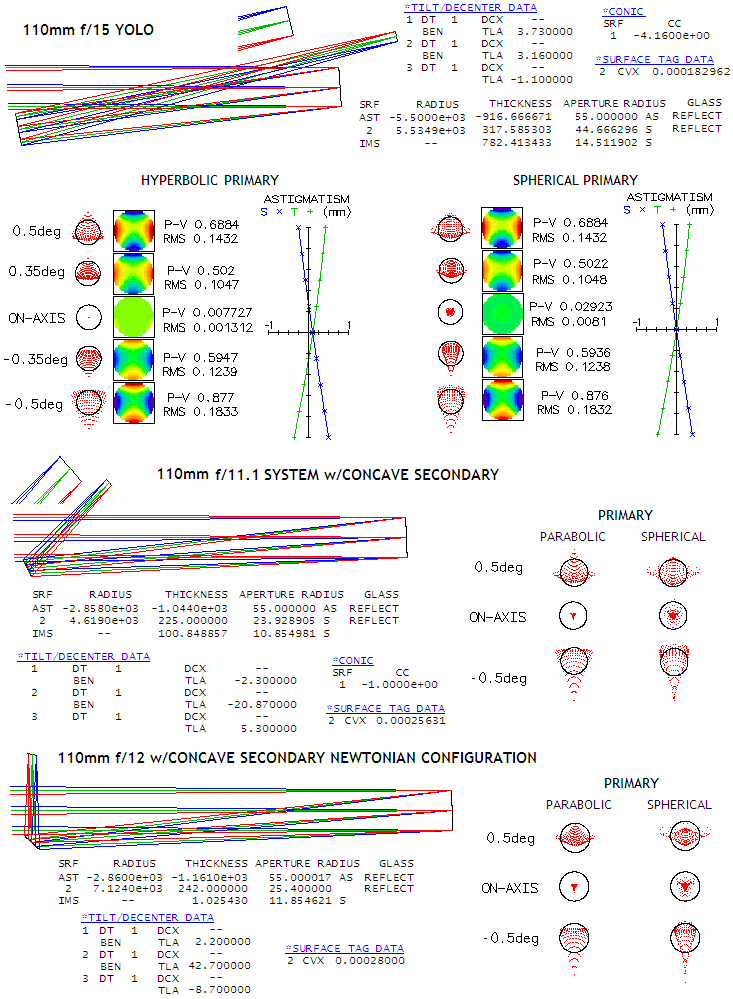The level of aberrations remains similar to the slower Yolo, mainly due to the less of primary tilt required. The primary is considerably faster, thus parabolizing it has more of an effect (secondary's contribution is negligible due to its small size), but it still doesn't make for appreciable difference: axial blur nearly the size of the Airy disc implies less than 1/12 wave P-V wavefront error. There is little difference in correction between arrangement w/tilted focusing cone (middle) and Newtonian-like configuration (bottom). While astigmatism can be always corrected, for any given secondary tilt angle there is only one secondary r.o.c. value at which this toroidal surface will also correct for axial coma. Off axis, there is some residual coma but, as wavefront maps show, astigmatism dominates. Trefoil is still significantly smaller than coma, thus only noticeable on axis (note that CVX in OSLO prescription stands for toric curvature, as the reciprocal of the radius value, not "convex").

These are the traditional two-mirror TCTs. Some of the most notable more recent developments in this field are described on the next page.